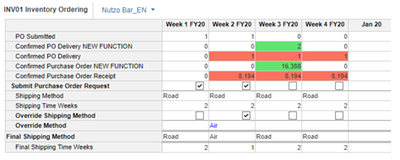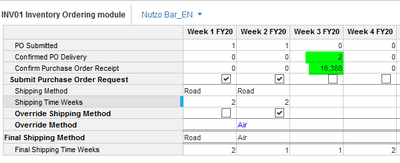# Level 2 Sprint 3 INV01Hi all,

So in building the INV01 Inventory Ordering module, I managed to get the same answer as the example given by the course module as follow:

Course Example:My Module:Unfortunately in my first attempt of exam, the formula for "Confirm Purchase Order Receipt" is incorrect, even though my answer is the same as the course example.

Do you know what may have done wrong here?

•I think your formula might not have been as per best practices. For example here are the two ways of writing the formula

IF Lineitem = 0  THEN X ELSE Y

IF Lineitem<> 0 THEN Y ELSE X

Output will be same but the calculation time by the engine will vary. Now the question is what to use when and the answer is - it depends

Logic is that your formula should exit as early as possible when satisfying the condition. Now I will leave upto to figure out where were you wrong? I am sure you will find out

Hope that helps